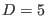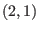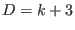# 1 Introduction

Topological field theories [1,2] are important in view of the fact that certain interacting, non-Abelian versions are related to a Poisson structure algebra  present in various versions of Poisson sigma models -, which are known to be useful at the study of two-dimensional gravity  - (for a detailed approach, see  ). It is well known that pure three-dimensional gravity is just a BF theory. Moreover, in higher dimensions general relativity and supergravity in Ashtekar formalism may also be formulated as topological BF theories with some extra constraints -. In view of these results, it is important to know the self-interactions in BF theories as well as the couplings between BF models and other theories. This problem has been considered in literature in relation with self-interactions in various classes of BF models - and couplings to other (matter or gauge) fields - by using the powerful BRST cohomological reformulation of the problem of constructing consistent interactions within the Lagrangian [39,40] or the Hamiltonian  setting, based on the computation of local BRST cohomology -. Other aspects concerning interacting, topological BF models can be found in  and .

On the other hand, tensor fields in `` exotic'' representations of the Lorentz group, characterized by a mixed Young symmetry type -, held the attention lately on some important issues, like the dual formulation of field theories of spin two or higher -, the impossibility of consistent cross-interactions in the dual formulation of linearized gravity , a Lagrangian first-order approach [63,64] to some classes of massless or partially massive mixed symmetry type tensor gauge fields, suggestively resembling to the tetrad formalism of General Relativity, or the derivation of some exotic gravitational interactions [65,66]. An important matter related to mixed symmetry type tensor fields is the study of their consistent interactions, among themselves as well as with other gauge theories -.

The purpose of this paper is to investigate the consistent interactions inbetween a massless tensor gauge field with the mixed symmetry of a two-column Young diagram of the typeand an Abelian BF model with a maximal field spectrum (a scalar field, two sorts of one-forms, two types of two-forms and a three-form). It is worth mentioning the duality of a free massless tensor gauge field with the mixed symmetryto the Pauli-Fierz theory indimensions. In view of this feature, we can state that our paper searches the consistent couplings inbetween the dual formulation of linearized gravity and a topological BF model. Our analysis relies on the deformation of the solution to the master equation by means of cohomological techniques with the help of the local BRST cohomology. We mention that the self-interactions in thesector have been investigated in  and the couplings inthat can be added to an Abelian BF model with a maximal field spectrum have been constructed in .

Under the hypotheses of analyticity in the coupling constant, spacetime locality, Lorentz covariance, and Poincaré invariance of the deformations, combined with the preservation of the number of derivatives on each field, we find a deformation of the solution to the master equation that provides nontrivial cross-couplings. The emerging Lagrangian action contains mixing-component terms of order one in the coupling constant that couple the massless tensor field with the mixed symmetrymainly to one of the two-forms and to the three-form from the BF sector. Also, it is interesting to note the appearance of some self-interactions in the BF sector at order two in the coupling constant that are strictly due to the presence of the tensor field with the mixed symmetry(they all vanish in its absence). The gauge transformations of all fields are deformed and, in addition, some of them include gauge parameters from the complementary sector. This is the first known case where the gauge transformations of the tensor field with the mixed symmetrydo change with respect to the free ones (by shifts in some of the BF gauge parameters). The gauge algebra and the reducibility structure of the coupled model are strongly modified during the deformation procedure, becoming open and respectively on-shell, by contrast to the free theory, whose gauge algebra is Abelian and the reducibility relations hold off-shell. Our result is important because dual formulations of linearized gravity have proved to be extremely rigid in allowing consistent interactions to themselves as well as to many matter or gauge theories. Actually, we think that this is the first time when a massless tensor field with the mixed symmetryallows consistent interactions that fulfill all the working hypotheses precisely in the dimensionwhere it becomes dual to the Pauli-Fierz theory.

Ashkbiz Danehkar
2018-03-26